Home | | Chemistry 12th Std | Answer the following questions

## Chapter: 12th Chemistry : UNIT 8 : Ionic Equilibrium

Chemistry : Ionic Equilibrium : Book Back Questions and Answers with Solutions: Answer the following questions

Chemistry : Ionic Equilibrium

1. What are Lewis acids and bases? Give two example for each.

ŌĆó A Lewis acid is a positive ion (or) an electron deficient molecule Example: BF3, CO2, Fe3+

ŌĆó A Lewis base is anion (or) neutral molecule with at least one lone pair of electrons

Example: NH3, FŌłÆ, Acetylene

2. Discuss the Lowry ŌĆō Bronsted concept of acids and bases.

ŌĆó An acid is defined as a substance that has a tendency to donate a proton to another substance (a proton donor)

ŌĆó A base is a substance that has a tendency to accept a proton form other substance (a proton acceptor)

ŌĆó Hydrogen chloride is dissolved in water, HCl behaves as an acid and H2O is base.

HCl + H2O Ōćī H3O+ + ClŌłÆ

Proton donar (acid) + Proton acceptor (Base) Ōćī Proton donar (acid) + Proton acceptor (Base)3. Indentify the conjugate acid base pair for the following reaction in aqueous solution

i) HS- (aq) + HF Ōåö F- (aq) + H2 S(aq)

ii) HPO42- + SO32- Ōåö PO43- + HSO3-

iii) NH4+ + CO32- Ōåö NH3 + HCO3

i) HSŌłÆ (aq) + HF Ōćī FŌłÆ(aq) + H2S(aq)

ii) HPO42ŌłÆ + SO32ŌłÆ Ōćī PO43ŌłÆ + HSO3ŌłÆ

iii) NH4+ + CO32ŌłÆ Ōćī NH3 + HCO3ŌłÆ

i) HSŌłÆ (aq) + HF Ōćī FŌłÆ (aq) + H2S(aq)ŌĆā

ŌĆó HF donates a proton to HSŌłÆ and gives FŌłÆ. HF is acid. Hence the conjugate base of HF is FŌłÆ.

ŌĆó HSŌłÆ accepts a proton from HF and forms H2S. HSŌłÆ is base and its conjugate acid is H2S.

ii) HPO42ŌłÆ + SO32ŌłÆ Ōćī PO43ŌłÆ + HSO3ŌłÆ

ŌĆó HPO42ŌłÆ donates a proton to SO32ŌłÆ. HPO42ŌłÆ is acid. Hence its conjugate base is PO43ŌłÆ

ŌĆó SO32ŌłÆ accepts a proton from HPO42ŌłÆ. SO32ŌłÆ is base. Hence its conjugate acid is HSOŌłÆ3.

iii) NH4+ + CO32ŌłÆ Ōćī NH3 + HCO3ŌłÆ

ŌĆó NH4+ donates a proton to CO32ŌłÆ. NH4+ is acid. Hence its conjugate base is NH3.

ŌĆó CO32ŌłÆ accepts a proton from NH4+. CO32ŌłÆ is base. Hence its conjugate acid is HCOŌłÆ3.

4. Account for the acidic nature of HClO4 in terms of Bronsted ŌĆō Lowry theory, identify its conjugate base.

ŌĆó HClO4 having the tendency to donate a proton. Hence it is acidic in nature.

ŌĆó HClO4ŌłÆ + H2O Ōćī H3O+ + ClO4ŌłÆ

perchloric acid + water Ōćī Hydronium ion + chlorate ion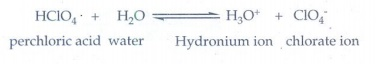ŌĆó HClO4 donates a proton to H2O and forms H3O+ and ClO4ŌłÆ

ŌĆó ClO4ŌłÆ is the conjugate base of HClO4.

5. When aqueous ammonia is added to CuSO4 solution, the solution turns deep blue due to the formation of tetramminecopper (II) complex, [Cu(H2 O)4 ](aq)2+ +4NH3 (aq) Ōåö [Cu(NH3)4 ](aq)2+ , among H2O and NH3 Which is stronger Lewis base.

Ammonia was added to the aqueous CuSO4 solution and forms tetramine Cu (II) complex.

Water present in the coordination sphere was replaced by NH3 and the complex formed. Moreover the higher electronegativity of oxygen also responsible for less availability of lone pair of electrons on the oxygen atom. [Hence NH3 is strong and Lewis base than H2O]

6. The concentration of hydroxide ion in a water sample is found to be 2.5 ├Ś10-6 M .. Identify the nature of the solution.

[OHŌłÆ] = 2.5 ├Ś 10-6 M

pOH = -Ōäōog (2.5 ├Ś 10ŌłÆ6)

= ŌłÆŌäōog102.5 + 6 Ōäōog10l0

= ŌłÆ0.3979 + 6 = 5.6021

pOH = 5.6021

pH + pOH = 14

pH = 14 ŌłÆ 5.6021 = 8.3979 = 8.4

Hence the solution is Basic

7. A lab assistant prepared a solution by adding a calculated quantity of HCl gas at 25oC to get a solution with [H3O+ ] = 4 ├Ś10-5M . Is the solution neutral (or) acidic (or) basic.

[H30+] = 4 ├Ś 10ŌłÆ5 M

pH = ŌłÆŌäōog [H3O+]

= ŌłÆlog (4 ├Ś l0ŌłÆ5)

= ŌłÆlog 4 + 5 log 10

pH = ŌłÆ0.6020 + 5 = 4.3979

pH = 4.4

The solution is acidic

8. Calculate the pH of 0.04 M HNO3 Solution.

Solution:

Concentration of HNO3 = 0.04M

[H3+] = 0.04 mol dm-3

pH = -log[H3O+]

= -log(0.04)

= -log(4 ├Ś10-2 )

= 2-log4

=2-0.6021

=1.3979 = 1.40

9. Define solubility product

The solubility product of a compound is defined as the product of the molar concentration of the constituent ions, each raised to the power of its stoichiometric co-efficient in a balanced equilibrium equation.10. Define ionic product of water. Give its value at room temperature.

2H2O Ōćī H3O+ + OHŌłÆ

According to law of mass action,

K eq = ( [H3O+] [OHŌłÆ] ) / ( [H2O]2)

Ōł┤ K eq = [H2O]2 = [H3O+] [OHŌłÆ]

Kw = l ├Ś 10ŌłÆ14 mol2 dmŌłÆ 6

The constant Kw is known as ionic product of water and it is given by the product of concentrations of [H30+] and [OHŌłÆ] ions at 298 K.

11. Explain common ion effect with an example

ŌĆó When a salt of a weak acid is added to the acid itself, the dissociation of the weak acid is suppressed further.

ŌĆó Example: Addition of sodium acetate to acetic acid solution.

ŌĆó The acetic acid dissociated weakly. The common ion, CH3COOŌłÆ suppress the dissociation of acetic acid

CH3COOH(aq) Ōćī H +(aq) + CH3 COOŌłÆ (aq)

acetic acid Ōćī Hydrogen ion + acetate ion

ŌĆó The added sodium acetate, salt completely dissociates to produce Na+ and CH3COOŌłÆ ion.

ŌĆó To maintain the equilibrium, the excess CH3COOŌłÆ ions combines with H+ ions to produce much more unionized CH3COOH and dissociation of CH3COOH suppressed.

12. Derive an expression for OstwaldŌĆÖs dilution law

Ostwald dilution law relates the dissociation constant of the weak electrolyte with degree of dissociation and the concentration of the weak electrolyte

CH3COOH Ōćī CH3COOŌłÆ + H+

Ka = ( [CH3 COOŌłÆ][H+] ) / [CH3COOH]

ŌłØ = degree of dissociation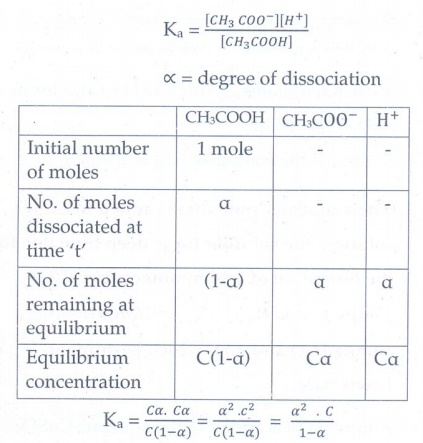Ka = C╬▒.C╬▒ / C(l-╬▒) = ╬▒2.c2 / C(lŌłÆ╬▒) = ╬▒2.C / lŌłÆ╬▒

If ╬▒ is too small Ka = ╬▒2c

Calculation for degree of dissociation (╬▒):

╬▒2 = kc/c , ╬▒ = ŌłÜ[ka/c]

Calculation for concentration:

[H+] = [CH3COOŌłÆ] = C╬▒

C. ŌłÜ[ka/c] = ŌłÜC . ŌłÜKa = ŌłÜ[Ka. C]

Ōł┤ Ka = ╬▒2C / 1ŌłÆ ╬▒ is known as the OstwaldŌĆÖs dilution law.

13. Define pH

pH of a solution is defined as the negative logarithm of base 10 of the molar concentration of the hydronium ions present in the solution.

pH = ŌłÆlog10[ H30+]

14. Calculate the pH of 1.5├Ś10-3M solution of Ba (OH)2

Ba(OH)2 ŌåÆ Ba2+ + 2OHŌłÆ

1.5 ├Ś 10ŌłÆ3 M ŌåÆ 2 ├Ś 1.5 ├Ś 10ŌłÆ3 M.

[OHŌłÆ] = 3 ├Ś 10ŌłÆ3M

[ŌłĄ pH + pOH = 14]

pH = 14 ŌłÆ pOH

pH = 14 ŌłÆ (ŌłÆlog[OHŌłÆ])

= 14 + log [OHŌłÆ]

= 14 + log (3 ├Ś l0ŌłÆ3)

= 14 + 0.4771ŌłÆ3

= 11 + 0.4771

pH = 11.48

15. 50ml of 0.05M HNO3 is added to 50ml of 0.025M KOH . Calculate the pH of the resultant solution.

Number of moles of HNO3 = 0.05 ├Ś 50 ├Ś l0ŌłÆ3

= 2.5 ├Ś 10ŌłÆ3

Number of moles of KOH = 0.025 ├Ś 50 ├Ś 10ŌłÆ3

= 1.25 ├Ś 10ŌłÆ3

Number of moles of HNO3 after mixing

= 2.5 ├Ś 10ŌłÆ3 ŌłÆ 1.25 ├Ś 10ŌłÆ3

= 1.25 ├Ś 10ŌłÆ3

ŌłĄ Concentration of HNO3

= Number of moles of HNO3 / Volume in litre

After mixing, total volume = 100 ml

= 100 ├Ś 10ŌłÆ3 L

ŌłĄ [H+] = (1.25 ├Ś 10ŌłÆ3 moles) / (100 ├Ś 10ŌłÆ3L)

= 1.25 ├Ś 10ŌłÆ2 moles LŌłÆ1

pH = ŌłÆlog [H+]

pH = ŌłÆlog10(1.25 ├Ś 10ŌłÆ2)

= ŌłÆlog10 l.25 + 21og10l0              [log10 l0 = l ]

pH = ŌłÆ0.0969 + 2 = 1.9031

16. The Ka value for HCN is 10-9 . What is the pH of 0.4M HCN solution?

Given:

Ka =10ŌłÆ9

c = 0.4 M

pH = ŌłÆlog[H+]

[H+] = ŌłÜ[Ka ├Ś c] = ŌłÜ[10-9 ├Ś 0.4] = 2 ├Ś 10-5

Ōł┤ pH = ŌłÆlog10(2 ├Śl0ŌłÆ5)

= log102 + 5 log10 10 = ŌłÆ0.3010 + 5 = 4.699

pH = 4.699

17. Calculate the extent of hydrolysis and the pH of 0.1 M ammonium acetate Given that Ka =Kb =1.8 ├Ś10-5

h = ŌłÜKh = ŌłÜ[ Kw / (KaKb) ] = ŌłÜ[(1x10ŌłÆ14)/(1.8 ├Ś 10-5 ├Ś 1.8 ├Ś 10- 5)]

= ŌłÜ [ (l/1.8) ├Ś 10-4 ] = ŌłÜ0.5555├Ś10-4

= 0.7453├Ś10-2

pH = 1/2 pKw + 1/2 PKa ŌĆō 1/2 PKb

Given that Ka = Kb = 1.8 ├Ś 10ŌłÆ5

If Ka = Kb , then pKa = pKb

Ōł┤ pH = l/2 pKw = 1/2 (14) = 7

18. Derive an expression for the hydrolysis constant and degree of hydrolysis of salt of strong acid and weak base

Let us consider the reactions between a strong acid, HCl, and a weak base, NH4OH, to produce a salt, NH4Cl and water

HCl(aq) + NH4OH(aq) Ōćī NH4Cl(aq)+H2O(Ōäō)

For strong acid

[H+] > [OHŌłÆ]; the solution is acidic and the pH is less than 7.

Kh.Kb = Kw

Kh = Kw/Kb

Kh = h2C

[H+] =ŌłÜ[Kh.C]

substitute Kh value in the above equation

[H+] = ŌłÜ{Kw/Kb . C}

pH = ŌłÆŌäōog10[H+]

= ŌłÆ log (Kw.C/ Kb)1/2

= ŌłÆ 1/2 log KwŌłÆ1/2 log C + 1/2 log Kb

PH = 7 - l/2pKb ŌłÆ l/2 log C

19. Solubility product of Ag2CrO4 is 1 ├Ś10-12 . What is the solubility of Ag2 CrO4 in 0.01M AgNO3 solution?

Given that Ksp =1 ├Ś 10-12

Ag2 CrO4 (s) Ōåö 2 Ag+(aq) + CrO4ŌłÆ2 (aq)

s Ōåö 2s + s

AgNO3 (s) Ōåö Ag+(aq) + NO3- (aq)

0.01M Ōåö 0.01M + 0.01M

[Ag+] = 2s + 0.01

0.01>>2S

Ōł┤ [Ag+ ]= 0.01M

[CrO42ŌłÆ]= s

Ksp = [Ag+]2 [CrO42- ]

1 ├Ś 10-12 = (0.01)2 (s)

(s) = 1 ├Ś 10-12 / (10-2)2 = 1 ├Ś 10ŌłÆ8 M

20. Write the expression for the solubility product of Ca3 (PO4 )2

Ca3(PO4)2 Ōćī 3Ca2+ + 2PO43-

s                    3s           2s

Ksp = [Ca2+]3 [PO43ŌłÆ]2

Ksp = (3s)3 (2s)2

Ksp = 27s3. 4s2

Ksp = 108 s5

21. A saturated solution, prepared by dissolving CaF2 (s) in water, has [Ca2+] = 3.3 ├Ś10-4M What is the Ksp of CaF2 ?

CaF2(s) Ōćī Ca(aq)2+ + 2FŌłÆ (aq)

[FŌłÆ] = 2 ├Ś 3.3 ├Ś 10-4 M

= 6.6 ├Ś 10ŌłÆ4 M

Ksp = [Ca2+][FŌłÆ]2

= (3.3 ├Ś 10ŌłÆ4) (6.6 ├Ś 10ŌłÆ4)2

= 1.44 ├Ś 10ŌłÆ10

22. Ksp of AgCl is 1.8 ├Ś10ŌłÆ10 . Calculate molar solubility in 1 M AgNO3

AgCl(s) Ōćī Ag+(aq) + ClŌłÆ (aq)

x = solubility of AgCl in 1M AgNO3

AgNO3(aq) Ōćī Ag+(aq) [1M] + NO3ŌłÆ (aq) [1M]

[Ag+] = x + 1       1M (ŌłĄ x < < 1)

[ClŌłÆ] = x

Ksp = [Ag+] [ClŌłÆ]

1.8 ├Ś 10ŌłÆ10 = (1) (x)

x = 1.8 ├Ś 10ŌłÆ10 M

23. A particular saturated solution of silver chromate Ag2 CrO4 has [Ag+ ]=5 ├Ś10-5 and [CrO4 ]2 -=4.4 ├Ś10 -4 M. What is the value of Ksp for Ag2 CrO4 ?

Ag2CrO4(s) Ōćī 2Ag+(aq) + CrO42ŌłÆ(aq)

Ksp = [Ag+]2 [CrO42ŌłÆ]

= (5 ├Ś l0-5)2 (4.4 ├Ś 10ŌłÆ4) = (25├Ś10ŌłÆ10) 4.4 ├Ś l0ŌłÆ4

= 1.1 ├Ś 10ŌłÆ12 = 110 ├Ś 10ŌłÆ14

= 1.10 ├Ś 10ŌłÆ12

24. Write the expression for the solubility product of Hg2 Cl2 .

Hg2Cl2 Ōćī Hg22+ + 2ClŌłÆ

s            s         2s

Ksp = [Hg22+] [ClŌłÆ]2

= (s) (2s)2

Ksp = 4s3

25. Ksp of Ag2 CrO4 is 1.1├Ś10-12 . what is solubility of Ag2 CrO4 in 0.1M K2 CrO4.

Ag2CrO4 Ōćī 2Ag+ + CrO42ŌłÆ

x                2x           x

x is the solubility of Ag2CrO4 in 0.1 M K2CrO4

K2CrO4 Ōćī 2K+ + CrO42ŌłÆ

0.1 M            0.2 M        0.1 M

[Ag+] = 2x

[CrO42ŌłÆ] = (x + 0.1) Ōēł 0.1          ŌłĄ x < < 0.1

Ksp = [Ag+]2 [CrO42ŌłÆ]

1.1 ├Ś 10ŌłÆ12 = (2x)2 (0.1)

1.1 ├Ś 10ŌłÆ12 = 0.4 x2

x2 = [1.1├Ś10ŌłÆ12] / 0.4 ; x = ŌłÜ[1.1 ├Ś 10ŌłÆ12] / [0.4]

x = ŌłÜ(2.75 ├Ś 10ŌłÆ12)

x = 1.65 ├Ś 10ŌłÆ6 M

26. Will a precipitate be formed when 0.150 L of 0.1M Pb(NO3)2 and 0.100L of 0.2 M NaCl are mixed? Ksp (PbCl2 )=1.2 ├Ś10-5 .

When two or more solutions are mixed, the resulting concentrations are different from the orignal.

Total volume = 0.150 L

Pb(NO3)2 Ōćī Pb2+ + 2NOŌłÆ3

0.1 M       0.1M      0.2 M

Number of moles

Pb2+ = molarity ├Ś Volume of the solution in liter

= 0.1 ├Ś 0.15

[Pb2+]mix = (0.1 ├Ś 0.15) / 0.25 = 0.06 M

NaCl    Ōćī   Na+     +    ClŌłÆ

0.2 M        0.2M    0.2M

No. of moles ClŌłÆ = 0.2 ├Ś 0.1

[ClŌłÆ]mix = (0.2 ├Ś 0.1) / 0.25 = 0.08 M

Precipitation of PbCl2 (s) occurs if

[Pb2+] [ClŌłÆ]2 > Ksp

[Pb2+] [ClŌłÆ]2 = (0.06) (0.08)2

= 3.84 ├Ś 10ŌłÆ4

Since ionic product [Pb2+] [ClŌłÆ]2 > Ksp , PbCl2 is precipitated

27. Ksp of Al(OH)3 is 1 ├Ś10-15M . At what pH does 1.0 ├Ś10-3M Al3+ precipitate on the addition of buffer of NH4Cl and NH4OH solution?

Al(OH)3 Ōćī Al3+(aq) + 3OHŌłÆ(aq)

Ksp = [A­ØæÖ3+] [OHŌłÆ]3

Al(OH)3 precipitates whenŌĆā

[Al3+] [OHŌłÆ]3 > Ksp

(l ├Ś10ŌłÆ3) [OHŌłÆ]3 > 1 ├Ś 10ŌłÆ15

[OHŌłÆ]3 > 1 ├Ś 10ŌłÆ12

[OHŌłÆ] > 1 ├Ś 10-4 M

[OHŌłÆ] = l├Ś 10-4 M

pOH = ŌłÆlog10[OHŌłÆ]= ŌłÆlog(1├Ś10ŌłÆ4) = 4

pH = 14 ŌłÆ 4 = 10

Thus, Al(OH)3 precipitates at a pH of 10

EVALUATE YOURSELF:

1. Classify the following as acid (or) base using Arrhenius concept

i) HNO3 ii) Ba(OH)2 iii) H3PO4 iv) CH3COOH

acid: i) HNO3 iii) H3PO4 iv) CH3COOH

base: ii) Ba(OH)2

2. Write a balanced equation for the dissociation of the following in water and identify the conjugate acid-base pairs.

i) NH4+ ii) H2SO4 iii) CH3COOH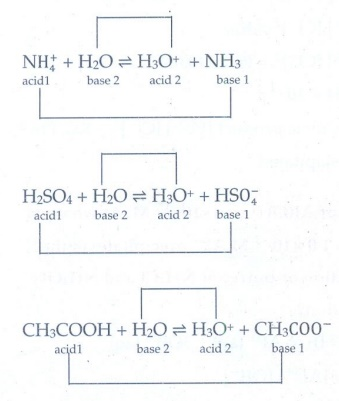NH4+   +       H2O      Ōćī       H3O+   +   NH3

acid l         base 2              acid 2      base 1

H2SO4 + H2O Ōćī H3O+ + HSO4-

acid l    base 2   acid 2   base 1

CH3COOH + H2O Ōćī H3O+ + CH3COO-

acid l     base 2    acid 2      base 1

3. Identify the Lewis acid and the Lewis base in the following reactions.

a) CaO + CO2 ŌĆö> CaCO3

CH3 Cl

b) CH3 - O - CH3 + AlCl3 ŌåÆi) CaO - Lewis base ; CO2 - Lewis acid

ii)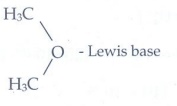AlCl3 - Lewis acid

4. H3BO3 accepts hydroxide ion from water as shown below

H3BO3(aq) + H2O(Ōäō) Ōćī B(OH)4- + H+

Predict the nature of H3BO3 using Lewis concept5. At a particular temperature, the Kw of a neutral solution was equal to 4xl0ŌłÆ14. Calculate the concentration of [H3O+] and [OH-].

Given solution is neutral

Ōł┤ [H3O+] = [OHŌłÆ]

Let [H3O+] = x; then [OHŌłÆ] = x

KW = [H3O+] [OHŌłÆ]

4 ├Ś 10ŌłÆ14 = x . x

x2 = 4 ├Ś 10ŌłÆ14

x = ŌłÜ[4├Ś10ŌłÆ14] = 2 ├Ś 10-7

6. a) Calculate pH of 10ŌłÆ8 H2SO4

b) Calculate the concentration of hydrogen ion in moles per litre of a solution whose pH is 5.4ŌĆā

c) Calculate the pH of an aqueous solution obtained by mixing 50 ml of 0.2 M HCl with 50ml 0.1 M NaOH.

a)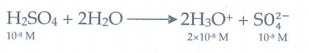In this case the concentration of H2SO4 is very low and hence [H3O+] from water cannot be neglected

Ōł┤ [H3O+] = 2 ├Ś 10ŌłÆ8 (from H2SO4) + 10-7 (from water)

= 10ŌłÆ8 (2 + 10)

= 12 ├Ś 10ŌłÆ8 = 1.2 ├Ś 10ŌłÆ7

pH = ŌłÆlog10[H3O+]

= ŌłÆlog10 (1.2 ├Ś l0ŌłÆ7)

= 7 ŌłÆ log10 1.2

pH = 7 ŌłÆ 0.0791 = 6.9209

b)

pH of the solution = 5.4

[H3O+] = antilog of (-pH)

= antilog of (ŌłÆ5.4)

= antilog of (ŌłÆ6 + 0.6)

= 3.981 ├Ś 10ŌłÆ6

[H3O+] = 3.98 ├Ś 10ŌłÆ6 mol dmŌłÆ3

c)

No of moles of HCl = 0.2 ├Ś 50 ├Ś 10ŌłÆ3 = 10 ├Ś l0ŌłÆ3

No of moles of NaOH = 0.1 ├Ś 50 ├Ś l0ŌłÆ3 = 5 ├Ś l0ŌłÆ3

No of moles of HCl after mixing

= (10 ├Ś l0ŌłÆ3 ) ŌĆō (5 ├Ś 10ŌłÆ3)

= 5 ├Ś 10ŌłÆ3

After mixing total volume = 100 mL

Ōł┤ Concentration of HCl in moles per litre

= [5 ├Ś 10ŌłÆ3 mole] / [100 ├Ś 10ŌłÆ3L]

[H3OŌłÆ] = 5 ├Ś 10ŌłÆ2 M

pH = ŌłÆlog10 (5 ├Ś l0ŌłÆ2) = ŌłÆlog105 + 21og1010

= 2 ŌłÆ log5 = 0.6990 + 2

= 2 ŌłÆ 0.6990 = 1.3010

= 1.30

pH = 1.3

7. Kb for NH4OH is 1.8 ├Ś 10-5. Calculating the percentage of ionisation of 0.06 M ammonium hydroxide solution.

╬▒ = ŌłÜ[Kb / C ] = ŌłÜ[1.8 ├Ś 10ŌłÆ5 ] / [ 6 ├Ś 10ŌłÆ2 ] = ŌłÜ(3 ├Ś 10-4)

= 1.732 ├Ś 10ŌłÆ2

= 1.732 / 100 = 1.732 %

8. a) Explain the buffer action in a basic buffer containing equimolar ammonium hydroxide and ammonium chloride.

b) Calculate the pH of a buffer solution consisting of 0.4M CH3COOH and 0.4M CH3COONa. What is the change in the pH after adding 0.01 mol of HCl to 500 ml of the above buffer solution. Assume that the addition of HCl causes negligible change in the volume.

Given:

(Ka = 1.8 ├Ś l0-5).

a) Dissociation of buffer components

NH4OH (aq) Ōćī NH4+ (aq) + OHŌłÆ (aq)

NH4Cl ŌåÆ NH4+ + ClŌłÆ

The added H+ ions are neutralized by NH4OH and there is no appreciable decrease in pH.

NH4OH(aq) + H+ ŌåÆ NH4+ (aq) + H2O (Ōäō)

The added OHŌłÆ ions react with NH4+ to produce unionized NH4OH. Since NH4OH is a weak base, there is no appreciable increase in pH

NH4+ (aq) + OHŌłÆ (aq) ŌåÆ NH4OH (aq)

b)

pH of buffer

CH3COOH (aq) Ōćī CH3COOŌłÆ (aq) + H+ (aq)

0.4 ŌĆō ╬▒                   ╬▒                     ╬▒

CH3COONa (aq) ŌåÆ CH3COOŌłÆ (aq) + Na+ (aq)

[H+] = Ka[CH3COOH] / [CH3C00ŌłÆ]

[CH3COOH] = 0.4 ŌłÆ ╬▒ Ōēā 0.4

[CH3COOŌłÆ] = 0.4 ŌłÆ ╬▒ Ōēā 0.4

Ōł┤ [H+] = Ka(0.4) / (0.4)

[H+] = 1.8 ├Ś 10-5

Ōł┤ pH = ŌłÆlog (1.8 ├Ś l0-5) = 4.74

Addition of 0.01 mol HCl to 500 ml of buffer

Added [H+] = 0.01 mol / 500 ml. = 0.01 mol / ┬Į L

= 0.02 M

CH3COOH(aq) Ōćī CH3COOŌłÆ (aq) + H+(aq)

0.4 - ╬▒                         ╬▒                    ╬▒

CH3COONa ŌåÆ CH3COOŌłÆ + Na+

0.4                      0.4            0.4

CH3COOŌłÆ + HCl ŌåÆ CH3COOH + C­ØæÖŌłÆ

(0.02)          0.02           0.02        0.02

Ōł┤ [CH3COOH] = 0.4 ŌłÆ ╬▒ + 0.02 = 0.42 ŌłÆ ╬▒ Ōēā 0.42

[CH3COOŌłÆ ] = 0.4 + ╬▒ ŌłÆ 0.02 = 0.38 + ╬▒ Ōēā 0.38

[H+] = [ (l.8 ├Ś l0-5) (0.42) ] / (0.38)

[H+] = 1.99 ├Ś 10-5

pH = ŌłÆlog (1.99 ├Ś 10-5)

= 5 ŌłÆ log 1.99

= 5 ŌłÆ 0.30 = 4.70

9. a) How can you prepare a buffer solution of pH 9. You are provided with 0.1M NH4OH solution and ammonium chloride crystals. (Given: pKa for NH4OH is 4.7 at 25┬░ C).

b) What volume of 0.6 M sodium formate solution is required to prepare a buffer solution of pH 4.0 by mixing it with 100ml of 0.8M formic acid. (Given: pKa for formic acid is 3.75).

a) pOH = pKb + log ( [salt] / [base] )

pH + pOH = 14

Ōł┤ 9 + pOH = 14

ŌćÆ pOH = 14 ŌłÆ 9 = 5

5 = 4.7 + log ( [NH4Cl] / [NH4OH] )

0.3 = log ( [NH4Cl] / 0.1)

[NH4Cl] / 0.1 = antilog of (0.3)

[NH4Cl] = 0.1 M ├Ś 1.995

= 0.1995 M

= 0.2 M

Amount of NH4Cl required to prepare 1 litre 0.2M solution

= strength of NH4Cl ├Ś molar mass of NH4Cl

= 0.2 ├Ś 53.5

= 10.70 g

10.70 g ammonium chloride is dissolved in water and the solution is made up of one litre to get 0.2 M solution. On mixing equal volume of the given NH4OH solution and the prepared NH4Cl solution will give a buffer solution with required pH value (pH = 9).

b)

PH = pKa + log ( [salt] / [base] )

4= 3.75 + log ([sodium formate] / [formic acid])

[sodium formate] = Number of moles of HCOONa / Volume of HCOONa

= 0.6 ├Ś V ├Ś 10ŌłÆ3

[Formic acid] = Number of moles of HCOOH / Volume of HCOOH

= 0.8 ├Ś 100 ├Ś 10ŌłÆ3

= 80 ├Ś 10ŌłÆ3

4 = 3.75 + log (0.6 V / 80)

0.25 = log (0.6 V / 80)

antilog of 0.25 = 0.6 V / 80

0.6 V = 1.778 ├Ś 80

= 142.4

V = 142.4 mL / 0.6 = 237.33 mL

10. Calculate the i) hydrolysis constant,

ii) degree of hydrolysis and iii) pH of 0.05M sodium carbonate solution pKa for HCOŌłÆ3 is 10.26.

Sodium carbonate is a salt of weak acid, H2CO3 and a strong base, NaOH and hence the solution is alkaline due to hydrolysis.

Na2CO3(aq) ŌåÆ 2Na+ (aq) + CO32ŌłÆ (aq)

CO32ŌłÆ (aq) + H2O(Ōäō) Ōćī HCO3ŌłÆ + OHŌłÆ

i) h = ŌłÜ[Kw / (Ka├ŚC) ]

= ŌłÜ(1 ├Ś 10ŌłÆ14) / (1.8 ├Ś 10 ŌłÆ5 ├Ś 0.1)

h = 7.5 ├Ś 10ŌłÆ5

Given that pKa = 4.74

pKa = ŌłÆlog Ka

ie., Ka = antilog of (ŌłÆpKa)

= antilog of (ŌłÆ4.74)

= antilog of (ŌłÆ5 + 0.26)

= 10ŌłÆ5 ├Ś 1.8

[antilog of 0.26 = 1.82 Ōēā1.8]

ii) Kh = Kw / Ka = 1├Ś10ŌłÆ14 / 1.8├Ś10-5

Kh = 5.56 ├Ś 10ŌłÆ10

iii) pH = 7 + pKa /2 + logC/2

pH = 7 + (4.74/2) + (log 0.1 / 2) = 7 + 2.37 ŌłÆ 0.5

= 8.87

Tags : Ionic Equilibrium | Chemistry , 12th Chemistry : UNIT 8 : Ionic Equilibrium
Study Material, Lecturing Notes, Assignment, Reference, Wiki description explanation, brief detail
12th Chemistry : UNIT 8 : Ionic Equilibrium : Answer the following questions | Ionic Equilibrium | Chemistry